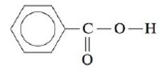Chapter 11, Problem 91AE

Chapter
Section
Textbook Problem

# The solubility of benzoic acid (HC7H5O2),is 0.34 g/100 mL in water at 25°C and is 10.0 g/100 mL in benzene (C6H6) at 25°C. Rationalize this solubility behavior. (Hint: Benzoic acid forms a dimer in benzene.) Would benzoic acid be more or less soluble in a 0.1-M NaOH solution than it is in water? Explain.

Interpretation Introduction

Interpretation: The solubility of Benzoic acid should be explained.

Concept Introduction:

Solubility:

• The solubility of the solute is depends on the many factors and the polarity of solute is one of that.
• The same polarity of solvent is favored the solubility of solute.
• The polarity of the compound is determined by its structure.
Explanation

Record data from given:

0.34 g/100 mL in water at 25°C10.0 g/100 mL in benzene at 25°C

To explain the solubility of benzoic acid in Water and Benzene.

Fig 1                                          Fig 2

• The benzoic acid is containing oxygen with lone pairs see Fig 1 and it is having electro negativity so it will make a hydrogen-bonded with another molecule to form a dimer

### Still sussing out bartleby?

Check out a sample textbook solution.

See a sample solution

#### The Solution to Your Study Problems

Bartleby provides explanations to thousands of textbook problems written by our experts, many with advanced degrees!

Get Started

#### Find more solutions based on key concepts